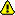Differences between revisions 16 and 18 (spanning 2 versions)
 ⇤ ← Revision 16 as of 2008-09-28 21:15:32 → Size: 2701 Editor: pong Comment: ← Revision 18 as of 2008-11-14 13:42:04 → ⇥ Size: 1312 Editor: localhost Comment: converted to 1.6 markup Deletions are marked like this. Additions are marked like this. Line 38: Line 38: http://moinmoin.wikiwikiweb.de/ [http://www.python.org/ Python] http://moinmoin.wikiwikiweb.de/ [[http://www.python.org/|Python]] Line 43: Line 43: http://c2.com/sig/wiki.gif {{http://c2.com/sig/wiki.gif}} Line 59: Line 59: drawing:mytest {{drawing:mytest}} Line 65: Line 65: == A graphical illustration of sin(x)/x -> 1 as x-> 0 ==by Wai Yan Pong{{{x=var('x')@interactdef _(x = slider(-7/10,7/10,1/20,1/2)):    html('

A graphical illustration of $\lim_{x -> 0} \sin(x)/x =1$

')    html('Below is the unit circle, so the length of the red line is |sin(x)|')    html('and the length of the blue line is |tan(x)| where x is the length of the arc.')     html('From the picture, we see that |sin(x)| $\le$ |x| $\le$ |tan(x)|.')    html('It follows easily from this that cos(x) $\le$ sin(x)/x $\le$ 1 when x is near 0.')    html('As $\lim_{x ->0} \cos(x) =1$, we conclude that $\lim_{x -> 0} \sin(x)/x =1$.')    if not (x == 0):        pretty_print("sin(x)/x = "+str(sin(float(x))/float(x)))    elif x == 0:        pretty_print("The limit of sin(x)/x as x tends to 0 is 1.")    C=circle((0,0),1, rgbcolor='black')    mvp = (cos(x),sin(x));tpt = (1, tan(x))    p1 = point(mvp, pointsize=30, rgbcolor='red'); p2 = point((1,0), pointsize=30, rgbcolor='red')    line1 = line([(0,0),tpt], rgbcolor='black'); line2 = line([(cos(x),0),mvp], rgbcolor='red')     line3 = line([(0,0),(1,0)], rgbcolor='black'); line4 = line([(1,0),tpt], rgbcolor='blue')    result = C+p1+p2+line1+line2+line3+line4    result.show(aspect_ratio=1, figsize=[3,3], axes=False)}}}attachment:sinelimit.png

Feel free to experiment here, after the four dashes below. Please do not create new pages without any meaningful content just to try it out!

You may want to open HelpOnEditing in a new window or tab while you edit.

## Formatting

italic bold typewriter

backtick typewriter (configurable)

bigger smaller

preformatted some more
and some more lines too

   1 def syntax(highlight):
2     print "on"
3     return None


   1   public void main(String[] args]){
2      System.out.println("Hello world!");
3   }## SmileysAlert

## Lists

### Bullet

• first
1. nested and numbered
2. numbered lists are renumbered
• second
• third blockquote
• deeper

Term
Definition